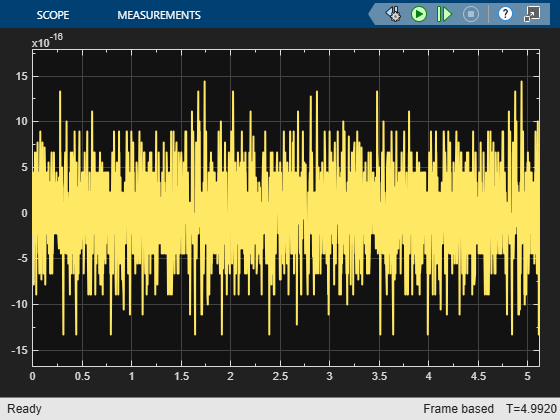# Transform Frequency-Domain Data into Time Domain

When you want to transform frequency-domain data into the time domain, use the IFFT block.

Use the Sine Wave block to generate two sinusoids, one at 15 Hz and the other at 40 Hz. Use a Matrix Sum block to add the sinusoids point-by-point to generate the compound sinusoid:Transform this sinusoid into the frequency domain using an FFT block, and then immediately transform the frequency-domain signal back to the time domain using the IFFT block. Plot the difference between the original time-domain signal and transformed time-domain signal using a scope:The two signals are identical to within round-off error. The scope shows that the difference between the two signals is on the order of.